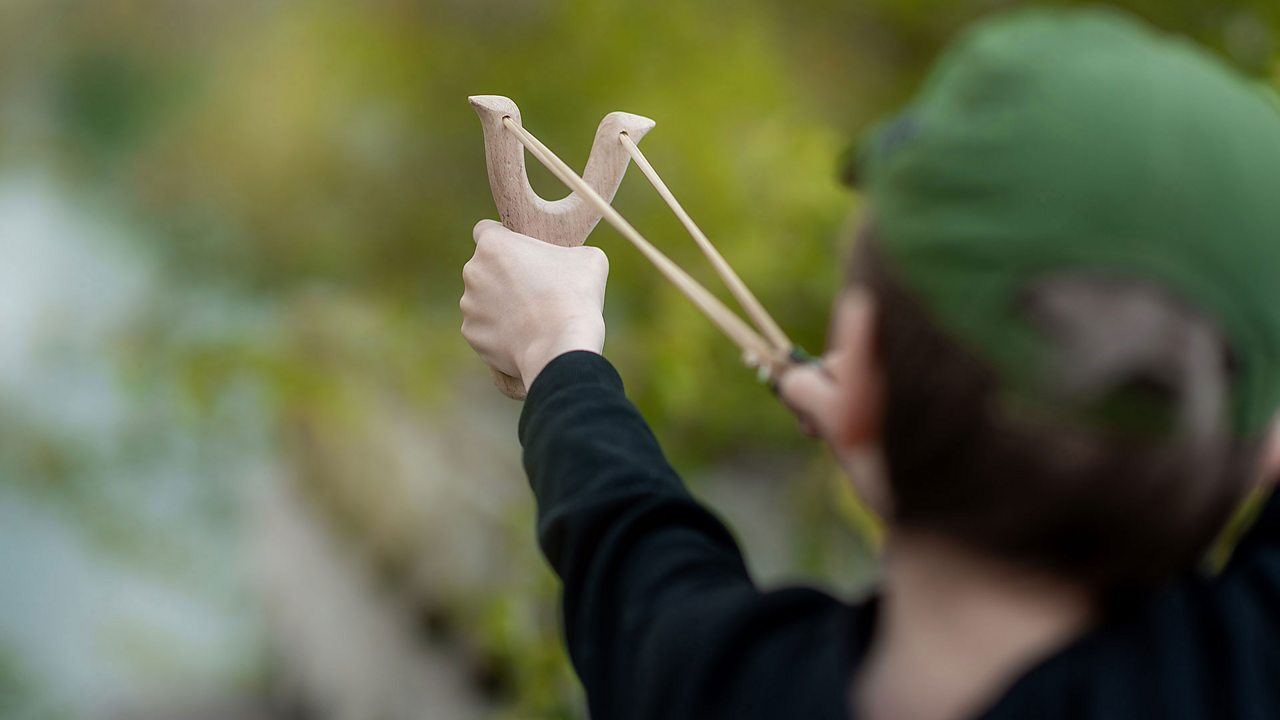# Energy stores and energy transfers

## Key points

• Energy can be described as being in different ‘stores’.

• Energy cannot be created or destroyed, but it can be transferred from one energy store to other energy stores.

• Some energy transfers are useful, but others are not.

## What is energy?

Energy is a very important idea in physics – it allows us to work out what might happen in a .

Energy is a helpful way to describe what has happened in a system, but it isn’t a very good way to explain why things happen. To explain why something happens, it is usually better to think about .

Energy can be a difficult idea to understand because we can’t see energy itself. One way we can understand energy is to use money as a model.

Money can be stored in different ways:

• in a bank account

• as bank notes in your wallet

• as coins in a piggy bank.

Money can be transferred from one store to another. Money doesn’t make things happen, but it does help to explain what might happen. For example, if you have a lot of money in your bank account, you could buy lots of expensive things.

Energy can also be stored in different stores, like the thermal store of a hot object, or the kinetic store of a moving object. The unit of energy is the (J).

## Energy stores

There are many different stores of energy.

Have a look at this slideshow to explore more about different stores of energy.The kinetic store of an object is filled when an object speeds up, and it empties when an object slows down1 of 5The gravitational store of an object is filled when an object is raised, and it empties when an object falls or is lowered2 of 5The elastic store of an object is filled when it is stretched or compressed3 of 5A chemical store is emptied during a chemical reaction when energy is transferred to the thermal store of the surroundings4 of 5The thermal store of an object is filled when a substance is heated, and it is emptied when the substance cools down5 of 5

## Energy transfers

It is helpful to describe how energy moves from one store to another during a process. Do this by choosing a start point (where you can identify a store of energy) and an end point.

Energy can be transferred from one store to another in four ways:

1. Mechanical work – a force is applied to move an object, for example when a person lifts a book onto a high shelf.
1. Electrical work – charges flow in the form of electricity, for example in a battery powered toy train.
1. Heat transfer – energy moves from the thermal store of a hotter object to the thermal store of a cooler object, for example when a handwarmer is used to warm up your hands.
1. Radiation - when energy is transferred as a wave, for example a grill cooking food using infra-red radiation.

## Work

When a force causes an object to move, work is being done. Work is a measure of the energy transferred when a force acts over a distance. This is often when a force moves an object, but work is also done when a force compresses or extends a spring or other flexible object.

This means that:

energy transferred = work

Work and energy are both measured in (J).

Work is not a store of energy - it is one of the ways in which energy can be transferred.

### Work done – equation

The following equation can be used to calculate work:

Work done in joules (J) = force in newtons (N) x distance moved in the direction of the force in metres (m)

$$W = F \times d$$

## How to calculate work

A boy lifts a toy dragon which weighs 30 newtons 2 metres above the ground. How much work did the boy do whilst lifting the dragon?

W = ?

F = 30 N

d = 2 m

The work done equation is:

$$W = F \times d$$

In this example:

$$W = 30 \times 2$$

The work done by the boy lifting the toy dragon is:

$$W = 60~J$$

## How to calculate the force applied

The equation for work done can be rearranged so that the force can be calculated if you know the values for work and distance.

The work done equation is:

$$W = F \times d$$

Divide both sides by distance:

$$\frac{W}{d} = \frac{F \times d}{d}$$

This cancels to give:

$$\frac{W}{d} = F$$

So to calculate the force, you must divide the work by the distance.

Have a look at this example:

What force is required to drag a heavy object 5 m if the work done is 4 000 J?

W = 4000 J

F = ?

d = 5 m

$$\frac{W}{d} = F$$

$$\frac{4000}{5} = F$$

$$800 = F$$

The force needed would be 800 newtons.

## How to calculate the distance moved

The equation for work can be rearranged to calculate the distance.

The work done equation is:

$$W = F \times d$$

Divide both sides by force:

$$\frac{W}{F} = \frac{F \times d}{F}$$

This cancels to give:

$$\frac{W}{F} = d$$

Have a look at this example:

How high can an object with a weight of 70 N be lifted if 210 J of work is done on it??

W = 210 J

F = 70 N

d = ?

$$\frac{W}{F} = d$$

$$\frac{210}{70} = d$$

$$3 = d$$

The object could be lifted 3 metres.